## Divide

To divide is to perform the operation of Division, i.e., to see how many times a Divisor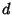goes into another number.divided byis writtenor. The result need not be an Integer, but if it is, some additional terminology is used.is readdivides'' and means thatis a Proper Divisor of. In this case,is said to be Divisible by. Clearly,and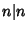. By convention,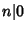for everyexcept 0 (Hardy and Wright 1979). The divided'' operation satisfiesis read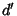does not divide'' and means thatis not a Proper Divisor of.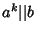means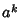dividesexactly.